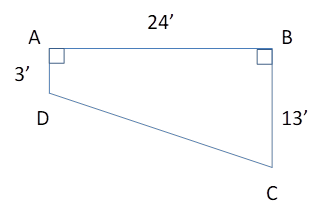Chapter 4.4, Problem 42EElementary Geometry For College St...

7th Edition
Alexander + 2 others
ISBN: 9781337614085

Solutions

Chapter
SectionElementary Geometry For College St...

7th Edition
Alexander + 2 others
ISBN: 9781337614085
Textbook Problem

For the in-ground pool shown in Exercise 41, find the length of the sloped bottom from point D to point C.To determine

To Find:

The length of the sloped bottom.

Explanation

Consider the following diagram.

Consider the triangle ΔABD.

According to Pythagoras theorem, (BD)2=(AB)2+(AD)2.

Now, (BD)2=(24)2+(3)2=576+9=585.

Therefore, BD=24

Still sussing out bartleby?

Check out a sample textbook solution.

See a sample solution

The Solution to Your Study Problems

Bartleby provides explanations to thousands of textbook problems written by our experts, many with advanced degrees!

Get Started

Find the limit or show that it does not exist. limx3x22x+1

Single Variable Calculus: Early Transcendentals, Volume I

Calculate y'. 7. y=t41t4+1

Calculus: Early Transcendentals

The general solution to dydt=ky is: a) y(t) = y(0)ekt b) y(t) = y(k)et c) y(t) = y(t)ek d) y(t) = ey(0)kt

Study Guide for Stewart's Single Variable Calculus: Early Transcendentals, 8th